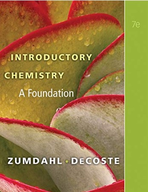Introductory Chemistry: A Foundation - 7 Edition - Chapter 2 - Problem 29
Register Now

Join StudySoup

Get Full Access to Introductory Chemistry: A Foundation - 7 Edition - Chapter 2 - Problem 299781439049402

# If you were to measure the width of this page using a ruler, and you used the ruler to

Introductory Chemistry: A Foundation | 7th Edition

Problem 29

If you were to measure the width of this page using a ruler, and you used the ruler to the limits of precision permitted by the scale on the ruler, the last digit you would write down for the measurement would be uncertain no matter how careful you were. Explain.

Accepted Solution
Step-by-Step Solution:
Step 1 of 3

▯ 13.1 & 13.4 Types of solutions: Inter-molecular Forces and Solubility ▯ ▯ Solubility & Equilibrium  Saturated: o A saturated solution is a type of solution that has maximum amount of dissolved SOLUTE when at a certain temperature.  When at that temperature it is in the presence of un- dissolved solute  Equilibrium: o Un-dissolved Solute = Dissolved Solute  H O o Ex: 2  MgBr Mg+ ( aq) + Cl-( aq) ▯  Unsaturated: o An unsaturated solution is a type of solution that has less than (

###### Chapter 2, Problem 29 is Solved

Step 2 of 3

Step 3 of 3

Unlock Textbook Solution